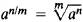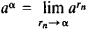# power

Also found in: Dictionary, Thesaurus, Medical, Legal, Financial, Acronyms, Idioms, Wikipedia.
Related to power: electric power, power series

## power

, in physics
power, in physics, time rate of doing work or of producing or expending energy. The unit of power based on the English units of measurement is the horsepower, devised for describing mechanical power by James Watt, who estimated that a horse can do 550 ft-lb of work per sec; a foot-pound is the work done when a weight (force) of 1 lb is moved through a distance of 1 ft. The unit of power in the metric system is the watt, named in honor of James Watt and equal to 1 joule per sec; the watt is used for measuring electric power in most countries, even those still using English units for other quantities. In common usage, the terms power and energy have become synonymous; for example, electrical energy is usually referred to as electric power (see power, electric). See also energy, sources of.

## Power

The time rate of doing work. Like work, power is a scalar quantity, that is, a quantity which has magnitude but no direction. Some units often used for the measurement of power are the watt (1 joule of work per second) and the horsepower (550 foot-pounds of work per second). See Work

Power is a concept which can be used to describe the operation of any system or device in which a flow of energy occurs. In many problems of apparatus design, the power, rather than the total work to be done, determines the size of the component used. Any device can do a large amount of work by performing for a long time at a low rate of power, that is, by doing work slowly. However, if a large amount of work must be done rapidly, a high-power device is needed. High-power machines are usually larger, more complicated, and more expensive than equipment which need operate only at low power. A motor which must lift a certain weight will have to be larger and more powerful if it lifts the weight rapidly than if it raises it slowly. An electrical resistor must be large in size if it is to convert electrical energy into heat at a high rate without being damaged.

McGraw-Hill Concise Encyclopedia of Physics. © 2002 by The McGraw-Hill Companies, Inc.

## power

The rate at which energy is expended by a source or work is done. It is measured in watts, i.e. in joules per second.
Collins Dictionary of Astronomy © Market House Books Ltd, 2006
The following article is from The Great Soviet Encyclopedia (1979). It might be outdated or ideologically biased.

## Power

a physical quantity measured as the ratio of work to the time interval during which the work is done. If the work is done at a uniform rate, power is defined by the formula N = A/t, where A is work done during the time t. In the general case N = dA/dt, where dA is the elementary work done during an elementary time interval dt (usually 1 sec). Power is measured in watts and sometimes (for engineering applications) in horse power.

## Power

Originally, the term “power” in arithmetic and algebra meant the product of a specified number of equal factors. The product of n equal factors a is denoted by a”. Here, a is called the base, and n is called the exponent. In English, the term “power” is sometimes used in the sense of “exponent.”

The second power of a, written a2, is also known as the square of a, or a squared. The third power of a, written a3, is known as the cube of a, or a cubed. It should be noted that a2 is the area of a square with sides of length a and that a3 is the volume of a cube with edges of length a.

The fundamental operations on powers are given by the following formulas:

 anm = an+m an ÷ am = an–m (an)m = anm

The concept of power came to be extended to cases where the exponent is not a positive integer. Thus, if the exponent is zero, we have a0 = 1 when a ≠ 0. Negative exponents are also possible: a–n = 1/an. For fractional exponents, we have(seeBINOMIAL EQUATION and EXTRACTION OF A ROOT). In the case of an irrational exponent α,where rn is an arbitrary sequence of rational numbers that approaches a. The rules of operations given above also hold for powers where the exponent is not a positive integer.

The theory of analytic functions deals with powers that have imaginary bases and exponents.

## power

[′pau̇·ər]
(mathematics)
The value that is assigned to a mathematical expression and its exponent.
The power of a set is its cardinality.
For a point, with reference to a circle, the quantity (x-a)2+ (y-b)2-r 2, where x and y are the coordinates of the point, a and b are the coordinates of the center of the circle, and r is the radius of the circle.
For a point, with reference to a sphere, the quantity (x-a)2+ (y-b)2+ (z-c)2-r 2, where x, y, and z are the coordinates of the point; a, b, and c are the coordinates of the center of the sphere; and r is the radius of the sphere.
(optics)
(physics)
The time rate of doing work.
(statistics)
One minus the probability that a given test causes the acceptance of the null hypothesis when it is false due to the validity of an alternative hypothesis; this is the same as the probability of rejecting the null hypothesis by the test when the alternative is true.
McGraw-Hill Dictionary of Scientific & Technical Terms, 6E, Copyright © 2003 by The McGraw-Hill Companies, Inc.

## power

The rate at which work is performed, energy is transformed or transferred, or energy is consumed; usually expressed in watts or horsepower.
McGraw-Hill Dictionary of Architecture and Construction. Copyright © 2003 by McGraw-Hill Companies, Inc.

## Power

Force, The
mystical source of a Jedi Knight’s righteous power. [Am. Cinema: Star Wars and sequels]

## power

1. control or dominion or a position of control, dominion, or authority
2. a state or other political entity with political, industrial, or military strength
3.
a. legal authority to act, esp in a specified capacity, for another
b. the document conferring such authority
4. Maths
a. the value of a number or quantity raised to some exponent
b. another name for exponent
5. Physics Engineering a measure of the rate of doing work expressed as the work done per unit time. It is measured in watts, horsepower, etc.
6.
a. the rate at which electrical energy is fed into or taken from a device or system. It is expressed, in a direct-current circuit, as the product of current and voltage and, in an alternating-current circuit, as the product of the effective values of the current and voltage and the cosine of the phase angle between them. It is measured in watts
b. (as modifier): a power amplifier
7.
a. mechanical energy as opposed to manual labour
b. (as modifier): a power mower
8.
a. a measure of the ability of a lens or optical system to magnify an object, equal to the reciprocal of the focal length. It is measured in dioptres
b. another word for magnification
9. the sixth of the nine orders into which the angels are traditionally divided in medieval angelology
Collins Discovery Encyclopedia, 1st edition © HarperCollins Publishers 2005

## POWER

Performance Optimization with Enhanced RISC. The IBM processor architecture on which PowerPC was based.

## power

(1) Electricity. See current, volt, watt, AC and DC.

(2) The capability of a computer system. See computer power and throughput.

(3) (POWER) (Performance Optimization With Enhanced RISC) A family of RISC-based CPUs from IBM. See POWER CPU and Power Systems.
Copyright © 1981-2019 by The Computer Language Company Inc. All Rights reserved. THIS DEFINITION IS FOR PERSONAL USE ONLY. All other reproduction is strictly prohibited without permission from the publisher.
References in periodicals archive ?
reserved to the states power over their internal economies.
CJ's opinion, that reservation of power was as clear as if it had
as the corporations power, which had to be interpreted so as to be
enabling the Commonwealth to invade the power over local trade which the
trade power reserved to the states unless the express words of the grant
required that the power extend to the internal affairs of the states.
Nuclear power plants take several hours to resume operation once they trip.
As we can see, the peacefulness of a power transition depends upon the satisfaction variable.
If the necessary power development is visible but a will to power is missing, then we are dealing with a power transition in the broader sense and could talk about an "eschewed" or a "missed" power transition.
The other constellations are not power transitions at all but should also be described.
All of the above have been quite in line with traditional PTT in that the final responsibility for the peaceful or nonpeaceful occurrence of a power transition rests solely on the shoulders of the rising power.
The role of the dominant power, in contrast, is rather passive and limited in standard PTT.

Site: Follow: Share:
Open / Close